# RS Aggarwal Solutions for Class 6 Chapter 4 Integers Exercise 4A

RS Aggarwal Solutions for Class 6, Chapter 4, Integers are available for students with the free download option. Solutions are created by BYJU’S faculty with a thorough explanation to help students with their exam preparation. These solutions are 100 percent accurate so that students can score more marks in the exams. Exercise 4A of RS Aggarwal Solutions has the questions based on methods of representing integers on the number line.

## Download PDF of RS Aggarwal Solutions for Class 6 Chapter 4 Integers Exercise 4A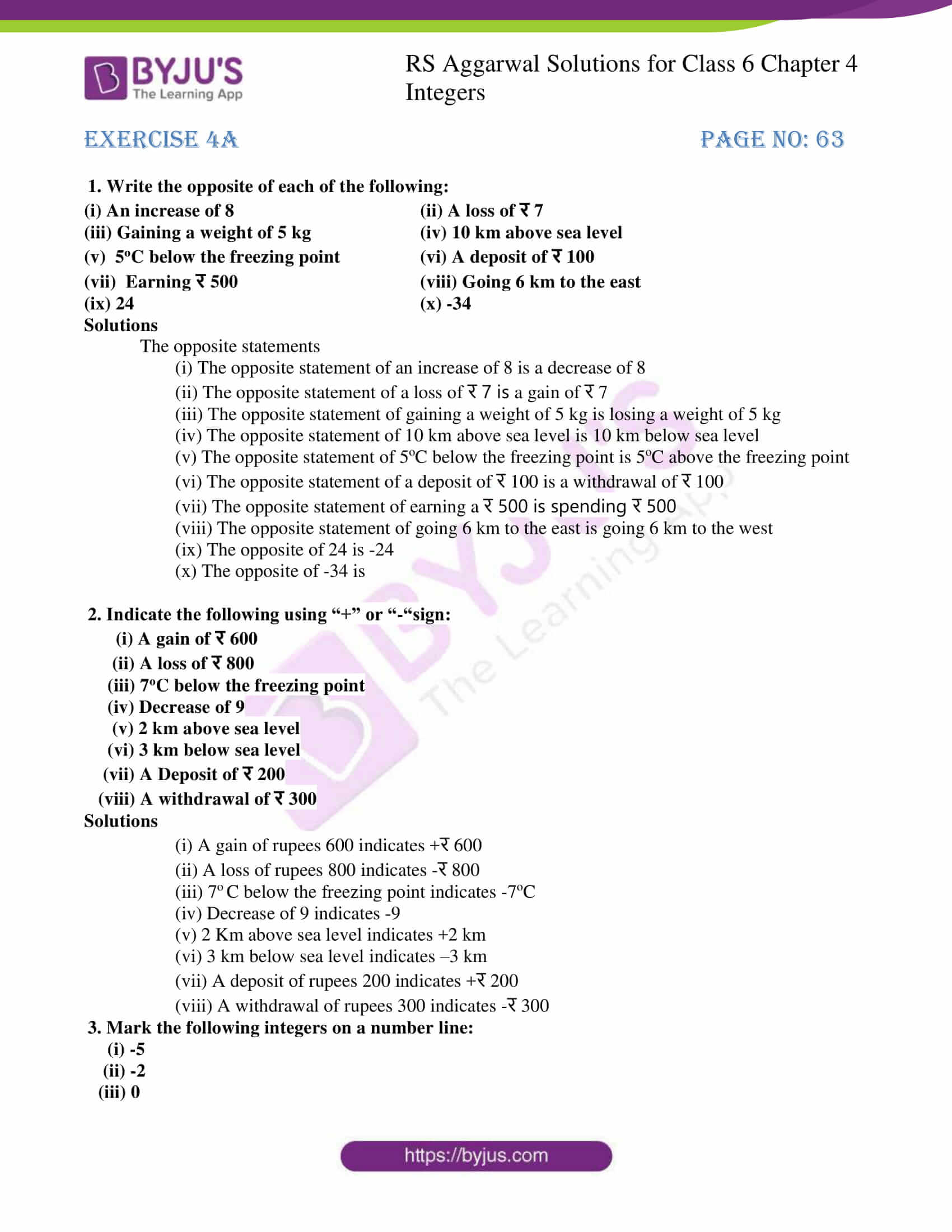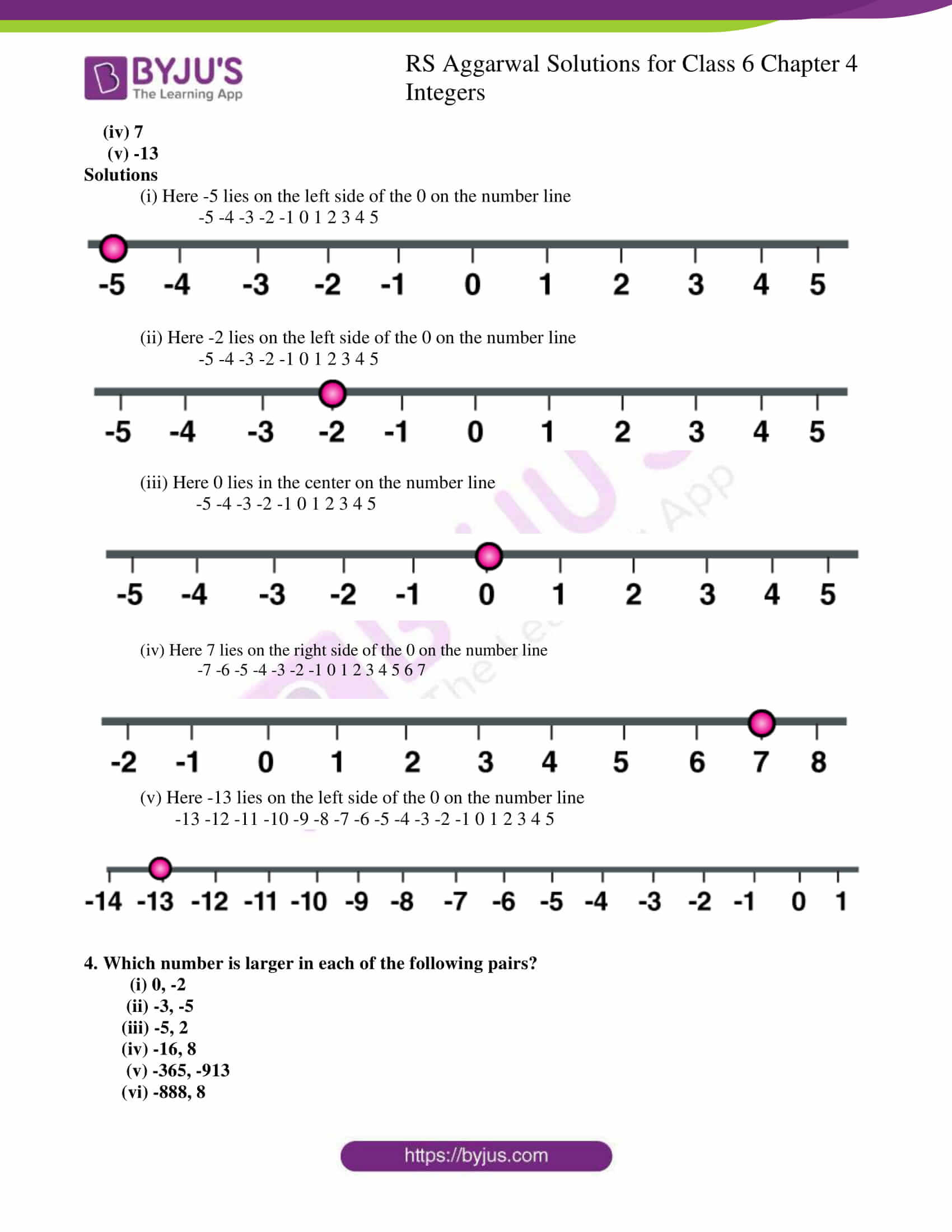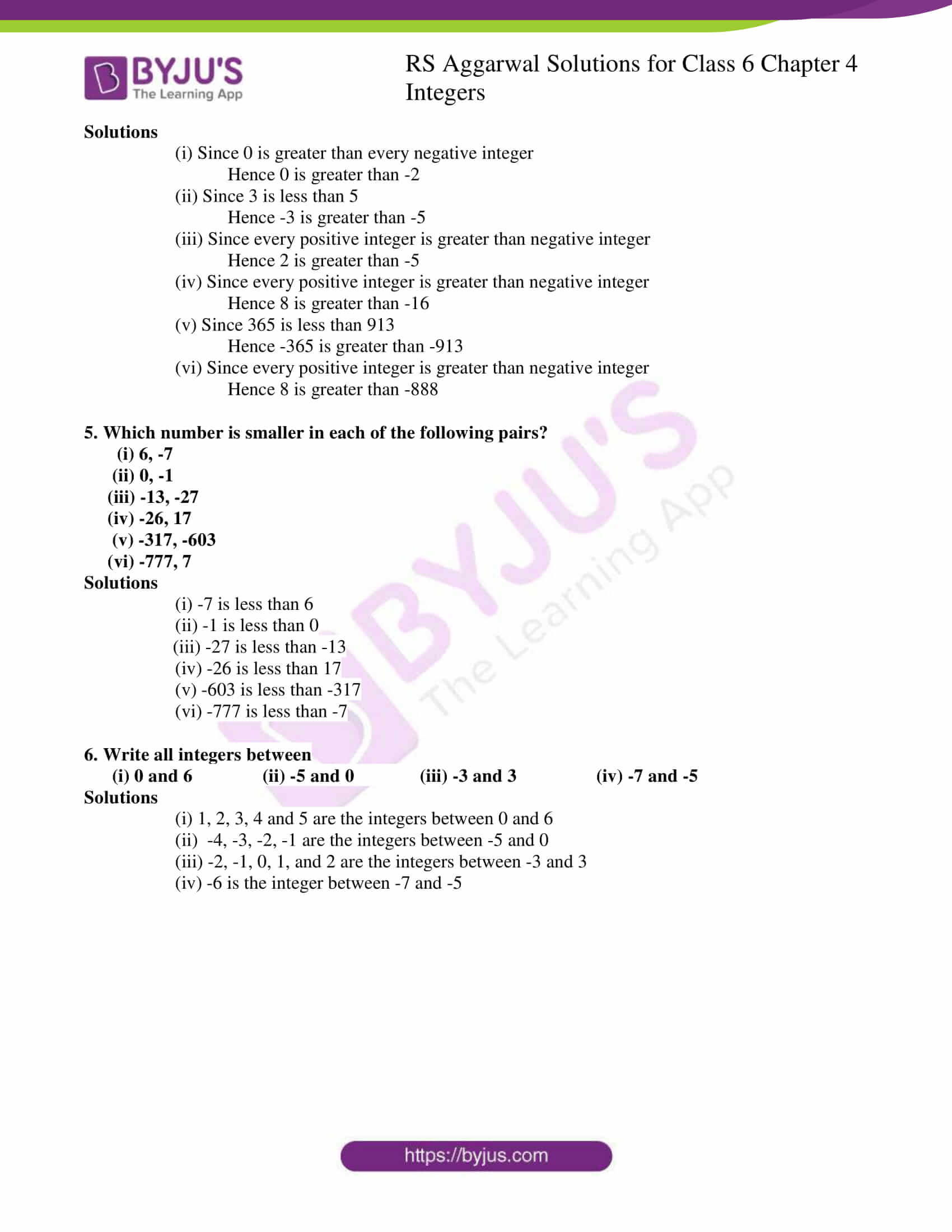## Access answers to Maths RS Aggarwal Solutions for Class 6 Chapter 4 Integers Exercise 4A

1. Write the opposite of each of the following:

(i) An increase of 8 (ii) A loss of र 7

(iii) Gaining a weight of 5 kg (iv) 10 km above sea level

(v) 5oC below the freezing point (vi) A deposit of र 100

(vii) Earning र 500 (viii) Going 6 km to the east

(ix) 24 (x) -34

Solutions

The opposite statements

(i) The opposite statement of an increase of 8 is a decrease of 8

(ii) The opposite statement of a loss of र 7 is a gain of र 7

(iii) The opposite statement of gaining a weight of 5 kg is losing a weight of 5 kg

(iv) The opposite statement of 10 km above sea level is 10 km below sea level

(v) The opposite statement of 5oC below the freezing point is 5oC above the freezing point

(vi) The opposite statement of a deposit of र 100 is a withdrawal of र 100

(vii) The opposite statement of earning a र 500 is spending र 500

(viii) The opposite statement of going 6 km to the east is going 6 km to the west

(ix) The opposite of 24 is -24

(x) The opposite of -34 is

2. Indicate the following using “+” or “-“sign:

(i) A gain of र 600

(ii) A loss of र 800

(iii) 7oC below the freezing point

(iv) Decrease of 9

(v) 2 km above sea level

(vi) 3 km below sea level

(vii) A Deposit of र 200

(viii) A withdrawal of र 300

Solutions

(i) A gain of rupees 600 indicates +र 600

(ii) A loss of rupees 800 indicates -र 800

(iii) 7o C below the freezing point indicates -7oC

(iv) Decrease of 9 indicates -9

(v) 2 Km above sea level indicates +2 km

(vi) 3 km below sea level indicates –3 km

(vii) A deposit of rupees 200 indicates +र 200

(viii) A withdrawal of rupees 300 indicates -र 300

3. Mark the following integers on a number line:

(i) -5

(ii) -2

(iii) 0

(iv) 7

(v) -13

Solutions

(i) Here -5 lies on the left side of the 0 on the number line

-5 -4 -3 -2 -1 0 1 2 3 4 5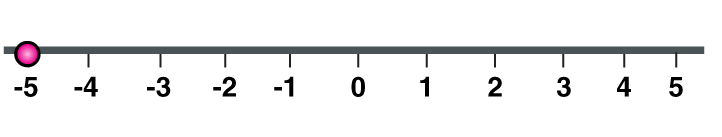(ii) Here -2 lies on the left side of the 0 on the number line

-5 -4 -3 -2 -1 0 1 2 3 4 5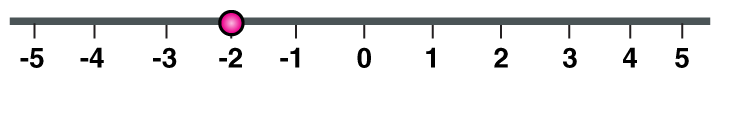(iii) Here 0 lies in the center on the number line

-5 -4 -3 -2 -1 0 1 2 3 4 5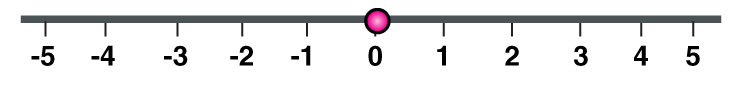(iv) Here 7 lies on the right side of the 0 on the number line

-7 -6 -5 -4 -3 -2 -1 0 1 2 3 4 5 6 7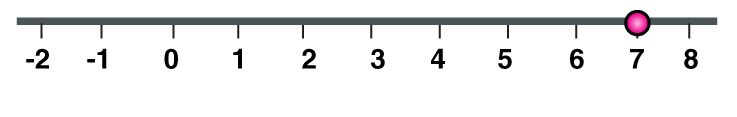(v) Here -13 lies on the left side of the 0 on the number line

-13 -12 -11 -10 -9 -8 -7 -6 -5 -4 -3 -2 -1 0 1 2 3 4 5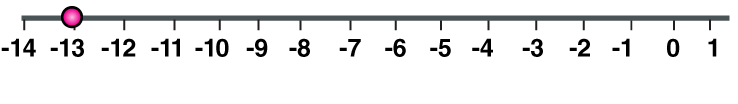4. Which number is larger in each of the following pairs?

(i) 0, -2

(ii) -3, -5

(iii) -5, 2

(iv) -16, 8

(v) -365, -913

(vi) -888, 8

Solutions

(i) Since 0 is greater than every negative integer

Hence 0 is greater than -2

(ii) Since 3 is less than 5

Hence -3 is greater than -5

(iii) Since every positive integer is greater than negative integer

Hence 2 is greater than -5

(iv) Since every positive integer is greater than negative integer

Hence 8 is greater than -16

(v) Since 365 is less than 913

Hence -365 is greater than -913

(vi) Since every positive integer is greater than negative integer

Hence 8 is greater than -888

5. Which number is smaller in each of the following pairs?

(i) 6, -7

(ii) 0, -1

(iii) -13, -27

(iv) -26, 17

(v) -317, -603

(vi) -777, 7

Solutions

(i) -7 is less than 6

(ii) -1 is less than 0

(iii) -27 is less than -13

(iv) -26 is less than 17

(v) -603 is less than -317

(vi) -777 is less than -7

6. Write all integers between

(i) 0 and 6 (ii) -5 and 0 (iii) -3 and 3 (iv) -7 and -5

Solutions

(i) 1, 2, 3, 4 and 5 are the integers between 0 and 6

(ii) -4, -3, -2, -1 are the integers between -5 and 0

(iii) -2, -1, 0, 1, and 2 are the integers between -3 and 3

(iv) -6 is the integer between -7 and -5